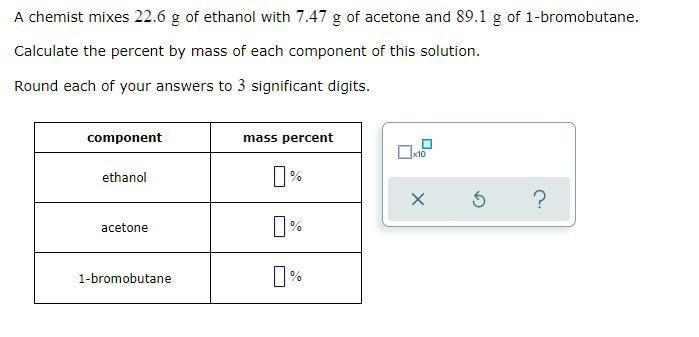Question:

# A chemist mixes 22.6 g of ethanol with 7.47 g of acetone and 89.1 g of 1-bromobutane. Calculate the percent by mass of each component of this solution. Round each of your answers to 3 significant digiA chemist mixes 22.6 g of ethanol with 7.47 g of acetone and 89.1 g of 1-bromobutane. Calculate the percent by mass of each component of this solution. Round each of your answers to 3 significant digits. component mass percent ethanol ? acetone ? 1-bromobutane ?